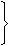# Aptitude - Chain Rule - Discussion

### Discussion :: Chain Rule - General Questions (Q.No.15)

15.

4 mat-weavers can weave 4 mats in 4 days. At the same rate, how many mats would be woven by 8 mat-weavers in 8 days?

 [A]. 4 [B]. 8 [C]. 12 [D]. 16

Explanation:

Let the required number of bottles be x.

More weavers, More mats (Direct Proportion)

More days, More mats (Direct Proportion)

 Wavers 4 : 8:: 4 : x Days 4 : 84 x 4 x x = 8 x 8 x 4x = (8 x 8 x 4) (4 x 4)x = 16.

 Neha said: (Aug 27, 2011) Give the explanation fully.

 Amitha said: (Sep 6, 2011) Please give a clear solution.

 No.7 said: (Sep 16, 2011) P M D 4 4 4 8 x 8.if .. x = 8*8*4/4*4 = 16 see...if no. of mats inc. then no. days also inc.(DIRECT) and if no. of people inc. then no. of days also inc.(DIRECT)

 Viraj Kamat said: (Nov 3, 2011) Consider this 4 mats in 4 days by 4 people Therefore each person weaves 1 mat in 4 days If there are 8 mat weavers and 8 days, each mat weaver can weave 2 mats in 8 days (1mat/4 days) Since there are 8 mat weavers : 8weavers * 2 mats = 16 mats !

 M.V.Krishna/Palvoncha said: (Jan 12, 2012) (IN 4 DAYS) 4 WEAVERS CAN WEAVE 4 MATS =>(1 WEAVER-1 MAT)--------(A) FROM (A): 8 WEAVERS-8 MATS SO 8 WEAVERS CAN WEAVE 8 MATS IN 4 DAYS. IF DAYS ARE DOUBLE, MATS WILL ALSO DOUBLE.(SINCE SAME RATE OF WORK AND NO CHANE IN WEAVERS) =>8 WEAVERS CAN WEAVE 16 MATS IN 8 DAYS.

 Moses said: (Apr 1, 2015) 4 weavers can weave 4 mats in 4 days. In one day one weaver weaves 1/4 of a mart at the same rate. Then 8 weavers weave 1/4 x 8 = 2 mats in one day. There fore in 8 days 8 weavers weave 8 x 2 =16 mats.

 M S Husain said: (Aug 29, 2015) It's too much simple. Here let x is a work. Chain Rule: (M1*D1)/W1 = (M2*D2)/W2. M-men or workers. D-Days or Time. W-Works. = (4*4)/4 = (8*8)/x. ==> x = 16.

 Prasanna said: (Nov 3, 2015) 4 mate weavers 4 days 4 mate. Which simply tells us that 4 mate weavers can make 1 mate/day. If mate weavers are 8 then 2 mates per day. So for 8 days 16 mats.

 Kriti said: (Jun 19, 2016) If 4 are weaving 4 mats in 4 days means 1 mat by 1 person then why not 8 men are weaving 8 mats in 8 days.

 Xyz said: (Sep 1, 2016) How it is solved? Please explain properly.

 Karthik said: (Sep 8, 2016) @XYZ Let's split the problem into 2 sides. * Mates per day. * Persons Per mate per day. In Problem it clearly states that 4 mates per 4 days. 4 mates --> 4 day. 1 mate ----> 4/4 = 1. So 4 mate weavers 1-day job = 1 mate. 8 mate weavers 1-day job = 1* 8/4 = 2 Mates. 8 mate weavers 2-day job = 2 * 8 = 16.

 Sangeetha said: (Apr 22, 2017) 4man can do 4 mats in 4 in 4 days. 1men can do 4 mats in 16 days. 1 man can do 1 mats in 4 days. 1 man can do 8 mats in 32 days. 8 man can do 8 mats in 4 days. So, 4 is my answer please explain me briefly that where have I done the mistake.

 Magnesh said: (Aug 31, 2017) Usually, we select answer as 8 because 4 4 4 so 8 8 8 actually we are wrong. 4 people 4 mats in 4 days. so 1 man can do 1 mat in 4 days. (If you think how 1 man 1mat in 4 days = effort of 4 people to make 1 mat in 1 day effort of 4 people to make 2 mats in 2day, similarly 4 people 4 mats 4 days as good as saying 1 man should work for 4 days to make 1 mat, because everyday he is putting effort to finish1/4 work of a mat, at the end of 4 days( 1/4+1/4+1/4+1/4= 1 mat )) Now, For simplicity keep days constant which is 4 days and people 8. 4 men make 4 mats in 4 days. 8 men make ? mats in 4 days. Since days are same don't worry and simply cross multiply. 8*4/4= 32/4= 8. So 8 mats in 4 days 8 mats in 4 days ? mats in 8 days 64 /4 = 16.

 Mp said: (Aug 31, 2017) @All. If A can complete a mat in 4 days. his 1 day of work is 1/4 so he completes 1/4 of mat in one day. 1/4 +1/4+1/4 +1/4 =1 mat.

 Sushi said: (Mar 10, 2019) @Viraj Kamat. Thank You.

 Minte said: (Aug 17, 2020) @All. Just think it like this, before 4 people complete 4 mats in 4 days, but with the addition of 4 people now 2 people can complete 2 mats in 1 day. So multiply the finished mats per day i.e 2 with the total no of days i.e 8 and we get 2* 8 = 16.

 Mani said: (Aug 22, 2020) Can any tell me, how to solve this by using direct and indirect proportions?

 Ponnu said: (May 29, 2021) M=4 M=8, D=4 D=8, H=x H=x, W=4 W=4. Weavers =4:8 Day's =4:8. Therefore, 4*4*x = 8*8*4 8*8*4 ÷ 4*4 x= 256 ÷16 = 16. So, The value if x = 16, Thanks.